# THERMAL PROPERTIES OF MATTER

### TEMPERATURE

The temperature of a body is that physical quantity which indicates how much hot or cold the body is? So, temperature indicates the hotness or coldness of a body.
The flow of heat is always from higher temperature to lower temperature. Temperature is a macroscopic concept.
Two bodies are said to be in thermal equilibrium with each other, when no heat flows from one body to the other, that is when both the bodies are at the same temperature.

TEMPERATURE MEASURING DEVICE
Thermometer is a device to measure the temperature. Thermometers used for measuring very high temperatures are called pyrometers.

DIFFERENT TYPES OF TEMPERATURE SCALES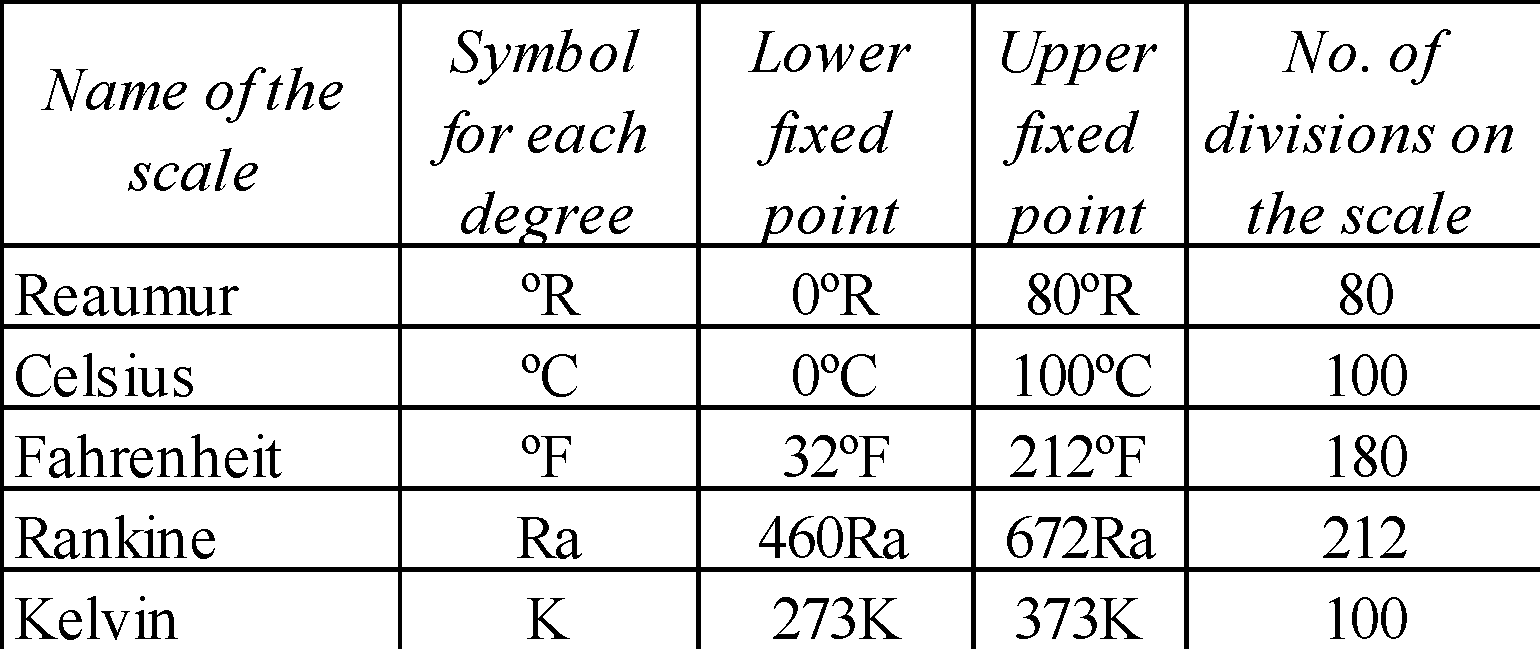• Lower fixed point (LFP) is the freezing point of water.
• Upper fixed point (UFP) is the boiling point of water.
TºK = (tºC + 273) or tºC = (TK – 273)

RELATIONSHIP BETWEEN DIFFERENT TEMPERATURE SCALESThe zero of the Kelvin scale is called absolute zero. The Kelvin scale is often termed as the absolute scale. In common use the absolute zero corresponds to –273ºC. However, its exact value is –273.16º C.

### IDEAL GAS EQUATION

The equation, PV = nRT
where, n = number of moles in the sample of gas
R = universal gas constant; (its value is 8.31 J mol–1 K–1), is known as ideal-gas equation
It is the combination of following three laws :
• Boyle's law : When temperature is held constant, the pressure is inversely proportional to volume.
i.e.,( at constant temperature)
• Charle's law : When the pressure is held constant, the volume of the gas is directly proportional to the absolute temperature.
i.e.,(at constant pressure)
• Avogadro's law :  When the pressure and temperature are kept constant, the volume is directly proportional to the number of moles of the ideal gas in the container.
i.e.,(at constant pressure and temperature)

### ABSOLUTE TEMPERATURE

The lowest temperature of –273.16 °C at which a gas is supposed to have zero volume and zero pressure and at which entire molecular motion stops is called absolute zero temperature. A new scale of temperature starting with –273.16°C by Lord Kelvin as zero. This is called Kelvin scale or absolute scale of temperature.
T(K) = t°C + 273.16
Thermometric property : It is the property used to measure the temperature. Any physical quantity such as length of mercury (in a glass capillary), pressure of a gas (at constant volume), electrical resistance (of a metal wire), thermo emf (thermocouple thermometer) which changes with a change in temperature can be used to measure temperature.
The unknown temperature t on a scale by utilising a general property X of the substance is given byKEEP IN MEMORY
1. The mercury thermometer with cylindrical bulb are more sensitive than those with spherical bulb.
2. Alcohol thermometer is preferred to the mercury thermometer due to the larger value of the coefficient of cubical expansion.
3. Properties that make mercury the ideal thermometric substance are :
1. It does not stick to the glass walls
2. Available in pure form
3. Low thermal conductivity and low specific heat
4. Vapour Pressure is low
5. Coefficient of expansion is uniform
6. It shines
4. Gas thermometer is more sensitive than the mercury thermometer, because its coefficient of cubical expansion is much larger.
5. At the following temperatures different temperature scales have the same reading :
1. Fahrenheit and Reaumur at –25.6ºF = –25.6ºR
2. Reaumur and Celsius at 0ºR = OºC
3. Celsius and Fahrenheit at –40ºC = –40ºF
4. Fahrenheit and Kelvin at 574.25ºF = 574.25K
6. The Celsius scale and the Kelvin cannot have the same reading at any temperature.
7. Six’s thermometer measures minimum and maximum temperature during a day. It uses mercury as well as alcohol as the thermometric substances.
8. Normal human body temperature is 37ºC = 98.6ºF.
9. Clinical thermometers are much shorter than the laboratory thermometers because they are used to measure a limited range (96º F to 100º F) of temperature.
10. Rapidly changing temperature is measured by using thermo electric thermometer.
11. The temperature inside a motor engine is measured using platinum resistance thermometer.
12. The radiation thermometers can measure temperature from a distance.
13. Adiabatic demagnetisation can be used to measure temperature very near to the absolute zero.
14. The Rankine is the smallest temperature range among all the scales of temperature.
15. When a substance is heated it expands along each dimension in the same proportion. (if it is uniform)

### THERMAL EXPANSION

#### THERMAL EXPANSION OF SUBSTANCES

LINEAR EXPANSION
i.e., increase in length with increase in temperatureorwhere l0 is the initial length, l = final length, ΔT = change in temperature, α = coefficient of linear expansion.

AREAL EXPANSION
i.e., increase in area with increase in temperature.where β = coefficient of area expansion

VOLUME EXPANSION
i.e. increase in volume with increase in temperature.where γ = coefficient of volume expansion. The units of α, β and γ is (°C)–1 or K–1.

Graph of αv versus T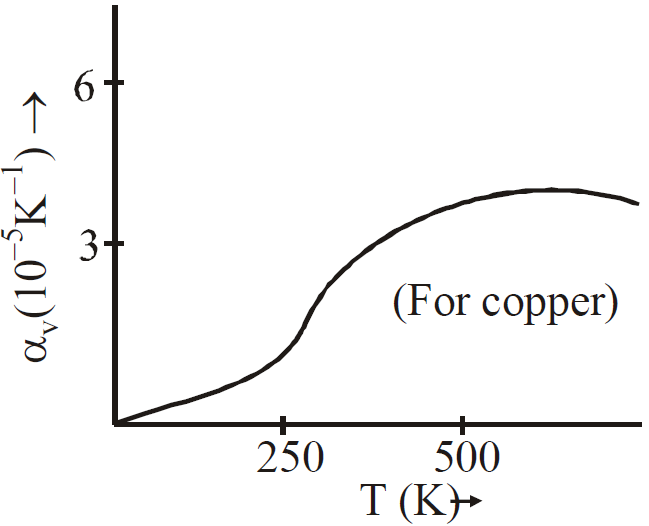KEEP IN MEMORY
1. During change in temperature, the mass does not change but the density decreases due to increase in volume. Therefore2. Relation between α, β and γand1. Water has negative coefficient of volume expansion for temperature range (0°C – 4°C). Therefore water contracts when the temperature rises from 0°C to 4°C and then expands as the temperature increases further.
Density of water reaches a maximum value of 1000 kg m–3 at 4°C. This is the anomalous behaviour of water.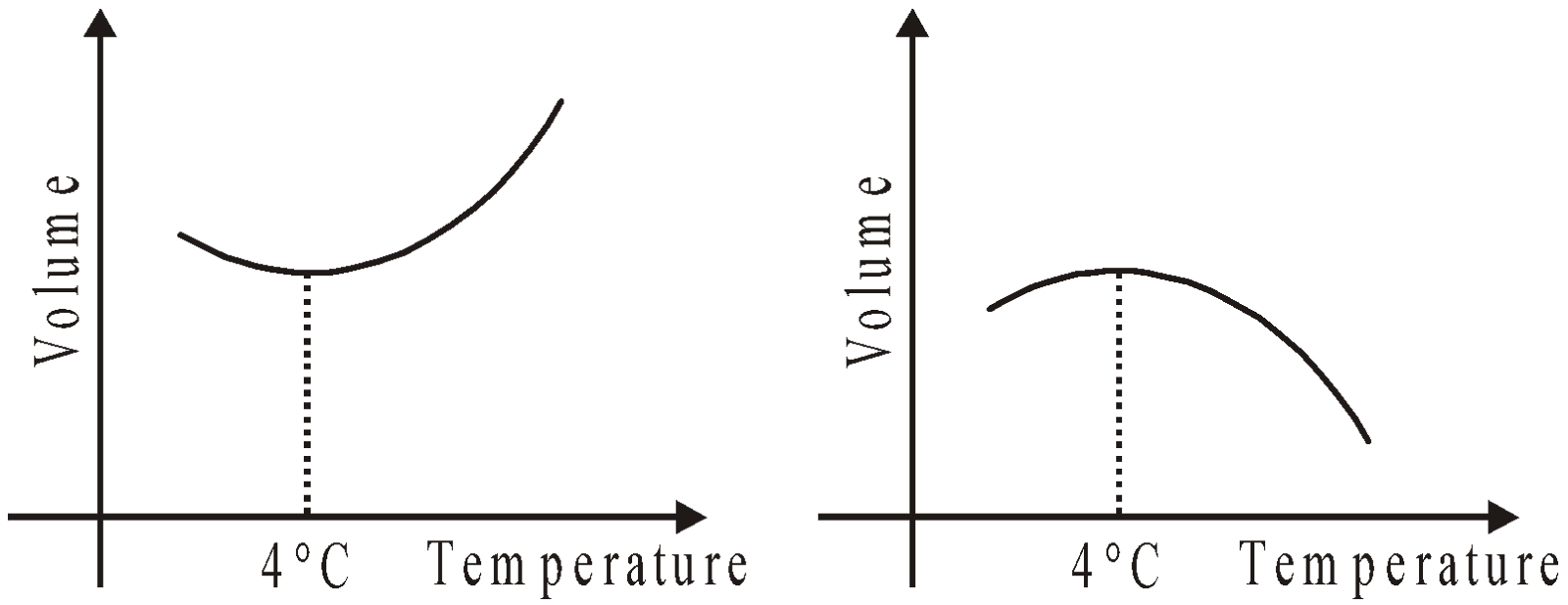#### THERMAL EXPANSION OF LIQUID (WHICH IS HEATED IN A VESSEL)where= apparent change in volume
V= volume of the liquid initially
γ = coefficient of volume expansion of liquid
α = coefficient of linear expansion of vessel

It is clear from the formula that
if,but
if,is positive and the liquid rises in the vessel and
if,is negative and liquid falls in vessel.

### CHANGE IN DENSITY OF SOLIDS AND LIQUIDS WITH TEMPERATURE

The density at t°C is given bywhere ρ0 is the density at 0°C. If ρ1 and ρ2 are the densities at temperature t1 and t2 respectively, then### THERMAL STRESS

If a rod fixed at two ends is heated or cooled, then stress are produced in the rod.
Thermal stress =### EFFECT OF TEMPERATURE  ON PENDULUM CLOCK

A Pendulum clock consists of a metal bar attached with a bob at one end and fixed at the other end. Let pendulum clock read correct time when its length is l0. The time periodSuppose that the temperature is raised by ΔT, then new time period is.....(i)
We have.....(ii)
From equations (i) and (ii),(since  is very small)so, fractional change in timeLoss of time per day( ⸪ no. of seconds in one day = 86400 sec.)
When temperature increases (during summer), the length of the pendulum increases due to which the time period increases and the clock loses time. On the other hand, when temperature decreases (during winter season), the length decreases and the time period decrease, the clock in this case, gains time.

As the temperature changes, the length of the metal scale changes so is the difference in graduation• Isothermal elasticity(since PV = RT = constant, );   where•### EXPANSION OF GASES

There are two coefficients of expansion of gases.
• Pressure coefficient of expansion of gas (γP)where P0 = Pressure at 0°C,  Pt = Pressure at t°C
• Volume coefficient of expansion of gas (γv)where V0 = Volume at 0°C, Vt = Volume at t°C

### HEAT

Heat is the form of energy which is transferred from one body to another body due to temperature difference between two bodies. Thermal energy of a body is the sum of kinetic energy of random motion of the molecules/atoms and the potential energy due to the interatomic forces.
Calorimeter is an instrument used to measure the heat.
The SI unit of heat is joule and its dimensions are [ML2T–2]. Other units of heat are :
• Calorie : It is the amount of heat required to raise the temperature of 1g of water from 14.5ºC to 15.5ºC.
1 calorie = 4.2 joule. Calorie is the unit of heat in CGS system.
• Kilocalorie, 1 Kcal = 1000 cal
• British Thermal Unit (BTU) : It is the fps unit of heat = 252 cal = amount of heat required to raise the temperature of 1 lb of water through 1ºF (from 63°F to 64ºF).

### SPECIFIC HEAT CAPACITY

It is the amount of heat required to raise the temperature of unit mass of substance through 1 degree.
Specific heat capacity,Its SI unit is J kg–1K–1
CGS unit cal g–1°C–1
It dimensions are [M0L2T–2 θ–1]

For gases :
During the isothermal process Δt = 0 ⇒ c = ∞ (infinite)
During the adiabatic process Q = 0  ⇒ c = 0 (zero)

Specific heat of gas : The specific heat of a gas varies from zero to infinity. It may have any positive or negative value.
Following of two specific heats of gas are more significant.
• Molar specific heat at constant volume (CV) : It is the amount of heat required to raise the temperature of 1 mole of a gas through 1K or 1°C at constant volume.• Molar specific heat at constant pressure (CP) : It is the amount of heat required to raise the temperature of 1 mole of a gas through 1K or 1°C at constant pressure.MAYER’S RELATION
CP – CV = R
[where R is a gas constant. (R = 8.314 Jmol–1K–1)]

Why CP is greater than CV?
When heat is supplied to a gas at constant volume entirely used to raise its temperature. When a gas is heated at constant pressure, some work is done in expansion of gas which is in addition to raise its temperature and hence CP > CV.

### THERMAL CAPACITY OR HEAT CAPACITY

It is the amount of heat required to raise the temperature of substance through 1ºC or 1K.
Thermal capacity = mass × specific heat
This is the relation between sp. heat capacity and heat capacity. Its SI unit is JK–1 CGS unit cal/°C. Its dimensions are [ML2T–2θ–1]

### WATER EQUIVALENT

It is the mass of water in gram which would require the same amount of heat to raise its temperature through 1ºC as the body when heated through the same temperature. It is measured in gram. Hence, Water equivalent of a body = mass of the body × specific heat

### PRINCIPLE OF HEAT (OR CALORIMETRY)

When two bodies at different temperatures are placed in contact with each other then heat will flow from the body at higher temperature to the body at lower temperature until both reach to a common temperature.
i.e. Heat lost by hot body = heat gained by cold body
It follows the law of conservation of energy.

### LATENT HEAT

It is defined as the amount of heat absorbed or given out by a body during the change of state while its temperature remaining constant.
It is of two types :
• Latent heat of fusion : It is the quantity of heat required to change unit mass of the solid into liquid at its melting point.
Latent heat of fusion of ice is 80 cal/g.
• Latent heat of vaporisation : It is the quantity of heat required to convert unit mass of liquid  into vapour at its boiling point.
Latent heat of vaporisation of water is 536 cal/g.
Latent heat increases the intermolecular potential energy of the molecules while kinetic energy remains constant.

### DIFFERENT PROCESSES OF PHASE CHANGE OR STATE CHANGE

• Sublimation : It is the process of conversion of solids to gaseous state on heating. On cooling the vapours get converted back into solids.
• Condensation : It is the process of conversion of gases/vapours to liquid state on cooling. On heating, these liquids are converted into vapours/gases.
• Boiling : It is the process of conversion of liquid to gaseous state on heating. It is a cooling process.
• Melting : It is the process in which solid gets converted into liquid on heating.
• Evaporation : Conversion of liquid into gaseous state at all temperatures is called evaporation. It is a phenomenon that occurs at the surface of the liquid.
Melting and boiling occur at definite temperature called melting point and boiling point respectively. The liquid boils at a temperature, at which its vapour pressure is equal to the atmospheric pressure.

How evaporation is different from boiling?
Evaporation is a slow process occurring at all the temperature at the surface of liquid while boiling is a fast process involving whole of the liquid heated to a particular temperature called boiling point of the liquid.

KEEP IN MEMORY
1. The heat capacity or thermal capacity depends on nature of the substance (specific heat capacity) and mass of quantity of matter of the body.
2. In case of melting and boiling, the temperature does not change, only intermolecular potential energy of the system changes.
3. System are in thermal equilibrium when their temperature are same or average kinetic energy per molecule is same.
4. Internal energy is the sum of all energies in a system which includes energy of translational, rotational as well as vibrational motion of the molecules.
5. The molar specific heat capacity depends on the molecular weight of the substance.
6. The temperature, volume or pressure of a system may remain constant when it absorbs heat.
7. When we press two block of ice together such that after releasing the pressure two block join this phenomenon is called regelation.

### PHASE DIAGRAM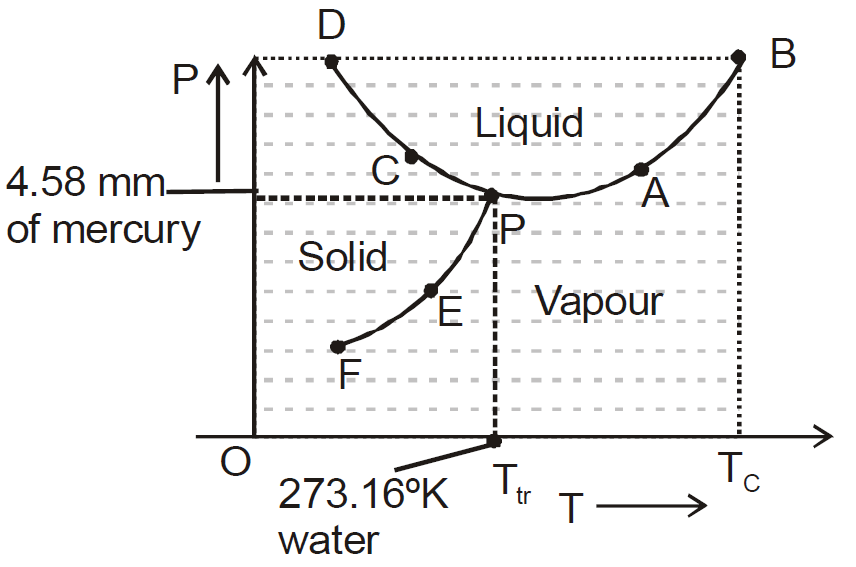• The diagram shows vaporisation curve (AB), fusion curve (CD), sublimation curve (EF).
• For water, AB is called steam line, CD is called ice line and EF is called hoar-frost line.
• The temperature at which all the three states of matter (solid, liquid, gas) are mutually in thermal equilibrium is called the triple point (point P in diagram) of the substance.
• The temperature upto which a gas can be liquified under pressure alone is called the critical temperature (Tc).

### SATURATED AND UNSATURATED AIR

The air is said to be saturated when the maximum possible amount of water vapours are present in it. The pressure of the water vapours in the saturated air is called saturation vapour pressure. If the air contains vapours less than the maximum possible amount possible in the air, then it (air) is said to be unsaturated.

### VAPOUR PRESSURE

A vapour differs from a gas in that the former can be liquefied by pressure alone, whereas the latter cannot be liquefied unless it is first cooled.

If a liquid is kept in a closed vessel, the space above the liquid becomes saturated with vapour. The pressure exerted by this vapour is called saturated vapour pressure. Its value depends only on temperature. It is independent of any external pressure.

### HUMIDITY

The humidity shows the presence of water vapours in the atmosphere. The amount of water vapours present per unit volume of the air is called absolute humidity.

RELATIVE HUMIDITY (RH) is defined as the ratio of the mass of water vapour (m) actually present in the given volume of air to the mass of water vapour (M) required to saturate the same volume at the same temperature. Normally RH is expressed in percentage
i.e.,RH is also defined as the ratio of actual pressure (p) of water vapour to the saturated vapour pressure (P) of the water at the same temperature
i.e.,Also,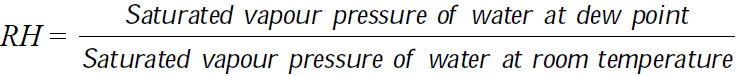DEW POINT
It is the temperature at which the amount of water vapours actually present in a certain volume of the air which is sufficient to saturate that volume of air.

At the dew point the actual vapour pressure becomes the saturated vapour pressure.
It means that vapour pressure at room temperature = saturated vapour pressure (S.V.P) at dew point

If room temperature = dew point
then Relative humidity (R.H.) = 100%

KEEP IN MEMORY
1. Hygrometry is the branch of thermal physics that deals with the measurement of amount of water vapours present in the atmosphere.
2. Hygrometer is an instrument used to determine dew point and relative humidity.
3. Relative humidity is low when the air is dry.
4. Saturated vapours do not obey (except Dalton’s law) gas laws, while unsaturated vapours obey them. The S.V.P. varies linearly with temperature (keeping volume constant) and equal to one atmospheric pressure at boiling point.(shown in fig.(a)) S.V.P. constant at constant temperature on increasing or decreasing volume fig. (b).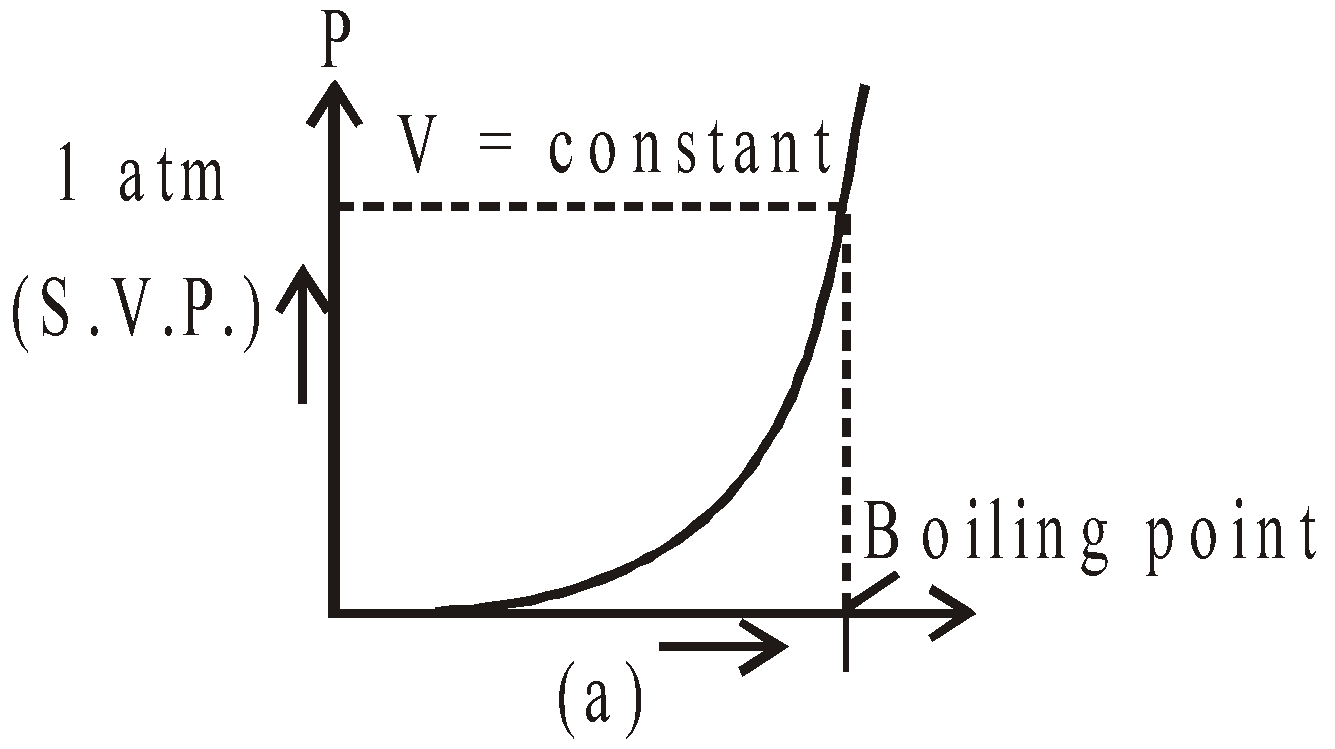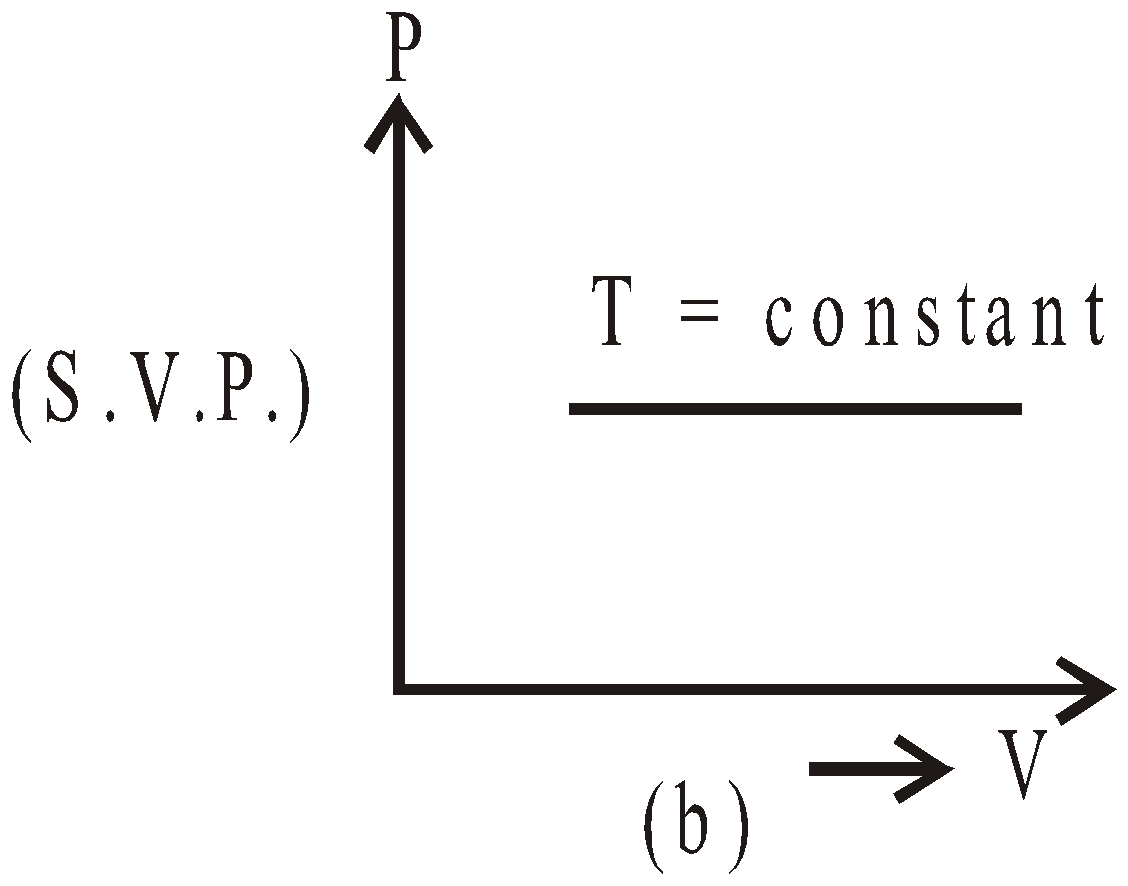1. At 0ºC, the saturation vapour pressure is 4.6 mm of Hg. Therefore, water vapours are always present around the ice. It increases with the increase in temperature.
2. The relative humidity is 100% when the temperature of the atmosphere is equal to the dew point.
3. Cryogenics is the study of low temperature.
4. As RH is expressed in percentage, hence the maximum value of RH may be 100%.
5. The saturation vapour pressure of water at 100ºC is 760 mm of Hg at sea level.
6. As the temperature rises, the absolute humidity may increase and the relative humidity may remain constant or may even decrease.
7. If the relative humidity is high then at the same temperature one feels hotter.
8. If the air is absolutely dry, the dew point is not observed.
9. The relative humidity decreases with the increase in temperature.
10. If water is sprinkled in the room, both relative humidity as well as the dew point increase.
11. Dew appears on the leaves of the trees etc. due to the condensation of saturated vapours.
12. The mist or fog is the small droplets of water formed due to the condensation of water vapours near the surface of earth.
13. Mist or fog formed much higher above the surface of earth is called cloud.
14. The Relative humidity between 50% and 60% gives comfortable feeling to human beings.
15. When the relative humidity is 100%, the reading of the dry and wet bulbs is same.
16. Smaller the difference in the temperature of dry and wet bulbs, larger is the relative humidity.
17. Water vapours are always seen around the ice, because the temperature around it is less than the dew point.

### HEAT TRANSFER

Three modes of transmission of heat :
• Conduction
• Convection

#### CONDUCTION

In thermal conduction particles of body at higher temperature transmit heat to the particles at lower temperature by mutual contact (collision) only and not by the movement of the particles. All solids are heated by conduction. Conduction cannot take place in vacuum.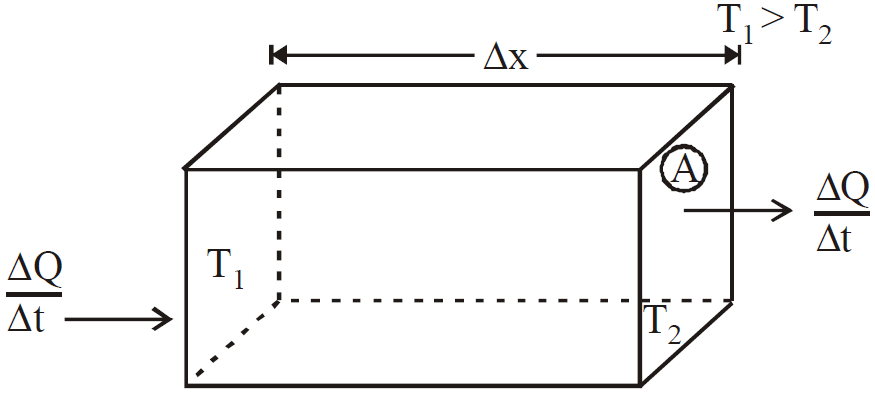Figure shows a solid of cross-section area A and thickness Δx. The face are at different temperature T1 and T2 (T1 > T2)
The rate of heat flowas found experimentally is given bywhere…(1)
where K is proportionality constant called thermal conductivity.
It is a measure of how quickly heat energy can conduct through the substance.

Coefficient of thermal conductivity (K) : The coefficient of thermal conductivity, K, of a material is defined as the amount of heat that  flowing per second through a rod of that material having unit length and unit area of cross-section in the steady state, when the difference of temperature between two ends of the rod is 1 ºC and flow of heat is perpendicular to the end faces of the rod.
Unit of coefficient of thermal conductivity in SI system is watt/m-K
Dimensions : [M L T–3 θ–1]

For a perfect conductor thermal conductivity K is infinite and for a perfect insulator K is zero.
In general, solids are better conductors than liquids and liquids are better conductors than gases. (Heat transfer through the process of conduction is possible in liquids and gases also, if they are heated from the top.) Metals are much better conductors than non-metals.
A good conductor of heat is also a good conductor of electricity. The conduction of both heat and electricity is due to the movement of free electrons.

#### CONVECTION

It is the process by which heat is transferred from one place to another in a medium by the movement of particles of the medium. It occurs due to density difference. This phenomenon occurs in fluids.

It is the process by which heat is transferred from one place to another without  any intervening medium. The light reaches from Sun to Earth by radiation process.
KEEP IN MEMORY
1. The equationis valid for steady state condition. The condition is said to occur when no part of heat is used up in raising the temperature of any part of cross-section of the solid.
2. On comparing equation (1) with the following equation used for flowing of charge on account of potential difference.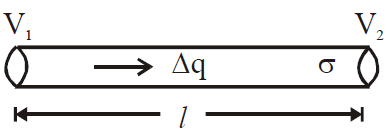We find :The role of resistance (thermal resistance) is played by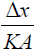1. Series combination of conductors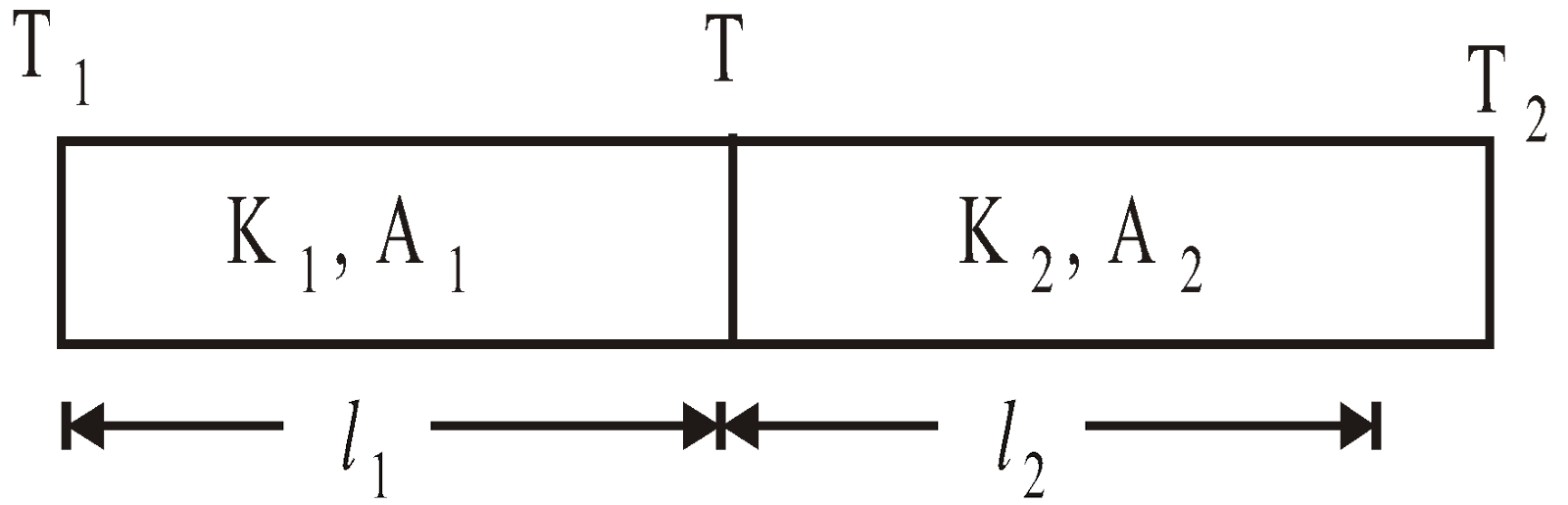Equivalent thermal conductivity :where H = heat flow per second
1. Parallel combination of conductors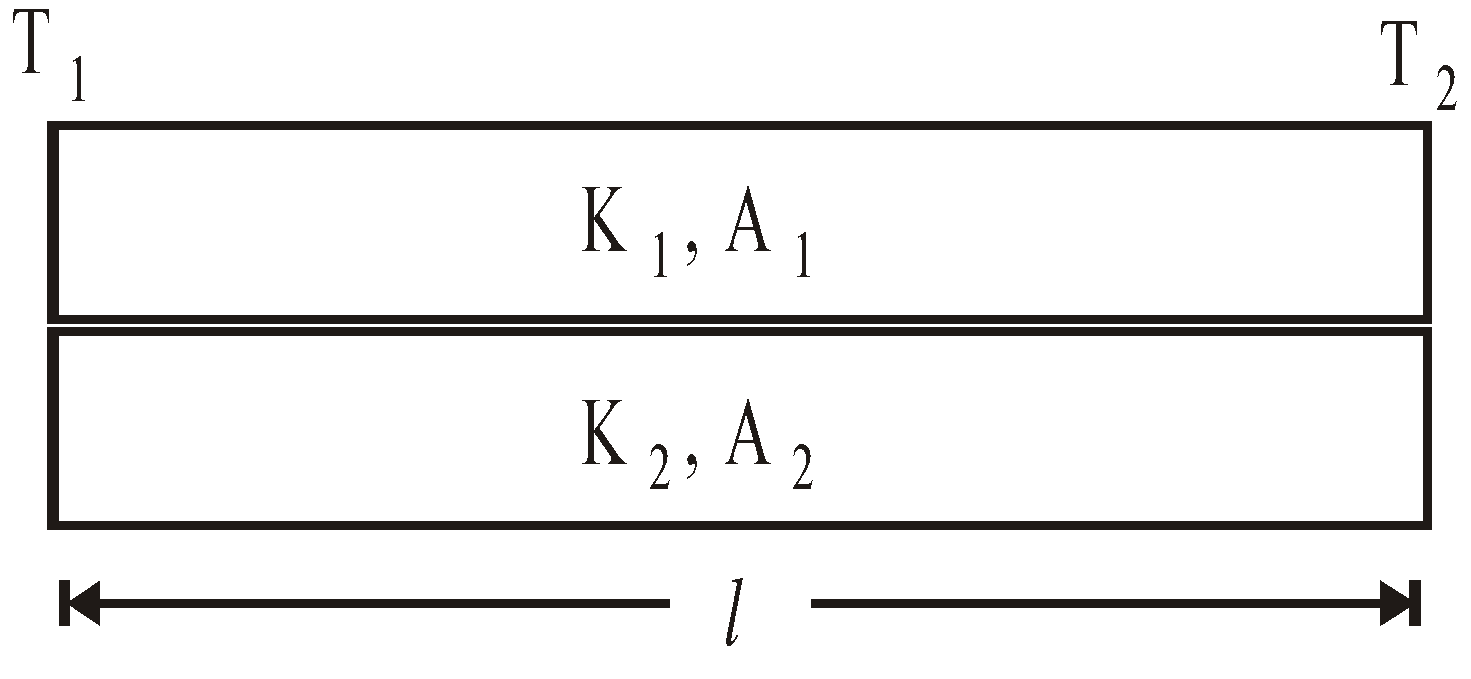Equivalent thermal conductivity :
Keq =1. Davy’s safety lamp is based on the conduction. It is used in mines to know the ignition temperature of gases. Danger of explosion can be avoided.
2. Principle of chimney used in a kitchen or a factory is based on the convection.
3. Land and sea breezes are due to the convection.
4. Temperature of the upper part of the flame is more than the temperature on the sides, because the currents of air carry the heat upwards.
5. Radiation can be detected by differential air thermometer, Bolometer, thermopile, etc.

### HEAT TRANSFER BY RADIATION AND NEWTON’S LAW OF COOLING

#### STEFAN’S LAW

This law is also called Stefan Boltzmann law. This law states that the power radiated for overall wavelength from a black body is proportional to the fourth power of thermodynamic temperature T.for perfectly black body ....(1)
where E is energy emitted per second from unit surface area of the black body, T is temperature and σ is Stefan’s constant, and
σ = 5.67×10–8 Wm–2 K–4.
If body is not perfectly black body, then
E = eσT4 ....(2)
where e is emissivity. Then energy radiated per second by a body of area A is
E1 = eσT4 ....(3)
If the body temperature is T and surrounding temperature is T0, then net rate of loss of energy by body through radiation from equation (3) is....(4)
Now rate of loss of energy in terms of specific heat c is....(5)
and....(6)
(T- temperature, t - time in second)
So body cools by radiation and rate of cooling depends on e (emissivity of body), A (area of cross-section of body), m (mass of body) and c (specific heat capacity of body).
A black body is defined as a body which completely absorbs all the heat radiation falling on it without reflecting and transmitting any of it.

#### WIEN'S LAW

λmT = b,
where b is the Wien's constant and b = 2.898 × 10–3mK
and T = temperature.

Graph of λm versus T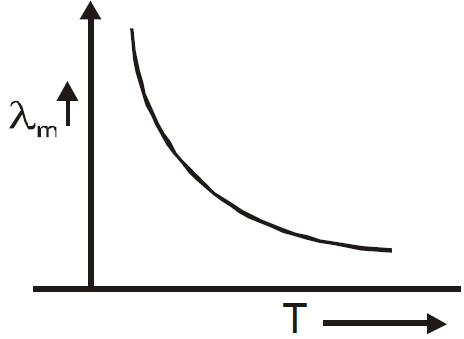Hence on increasing temperature of a body, its colour changes gradually from redorangeyellowgreenblueviolet. Thus the temperature of violet star is maximum and temperature of red star is minimum. Sun is a medium category star with λm = 4753Aº (yellow colour) and temperature 6000K.

#### KIRCHOFF’S LAW

According to this law, the ratio of emissive power to absorptive power is same for all surfaces at the same temperature and is equal to the emissive power of a perfectly black body at the same temperature.
i.e.where e = emissive power of a given surface
a = absorptive power of a given surface
E = emissive power of a perfect black body
A = absorptive power of a perfect black body
• For a perfect black body, A = 1,• If emissive and absorptive power are considered for a particular wavelength λ then• Since Eλ is constant at a given temperature, according to this law if a surface is good absorber of a particular wavelength then it is also a good emitter of that wavelength.

#### FRAUNHOFER'S LINE

• Fraunhofer lines are the dark lines in the spectrum of sun and are explained on the basis of Kirchoff’s law. When white light emitted from central core of sun (photosphere) passes through its atmosphere (chromosphere) radiation of those wavelength will be absorbed by the gases present there, which they usually emit (as good emitter is a good absorber) resulting dark lines in the spectrum of sun.
• On the basis of Kirchoff’s law, Fraunhofer identified some of the elements present in the chromosphere. They are hydrogen, helium, sodium, iron, calcium, etc. Fraunhofer had observed about 600 darklines in the spectrum of sun.

#### NEWTON'S LAW OF COOLING

The rate of loss of heat of a body is directly proportional to the temperature difference between the body and the surroundings.
i.e.where T = temperature of body, T0 = temperature of surrounding.
andwhere, e = emissivity of body, A = area of surface of body, σ = Stefan’s constant, m = mass of body, c = specific heat of body.

It is a special case of Stefan’s law and above relation is applicable only when the temperature of body is not much different from the temperature of surroundings.

#### SOLAR CONSTANT

Solar constant is the solar radiation incident normally per second on one square metre at the mean distance of the earth from the sun in free space.
It is given by S = 1.34 × 109 Jm–2s–1.
Temperature of sun is given T4 = S/σ(R/r)2, where R is mean distance of the earth from the sun and r is the radius of the sun.

KEEP IN MEMORY
• Good absorber is a good emitter.
• Cooking utensils are provided with wooden or ebonite handles, since wood or ebonite is a bad conductor of heat.
• Good conductor of heat are good conductors of electricity, Mica is an exception which being a good conductor of heat is a bad conductor of electricity.## Want to know more

Want to Know More
Please fill in the details below:

## Latest NEET Articles\$type=three\$c=3\$author=hide\$comment=hide\$rm=hide\$date=hide\$snippet=hide

Name

ltr
item
BEST NEET COACHING CENTER | BEST IIT JEE COACHING INSTITUTE | BEST NEET, IIT JEE COACHING INSTITUTE: Thermal Properties of Matter | Physics Notes for IITJEE/NEET
Thermal Properties of Matter | Physics Notes for IITJEE/NEET